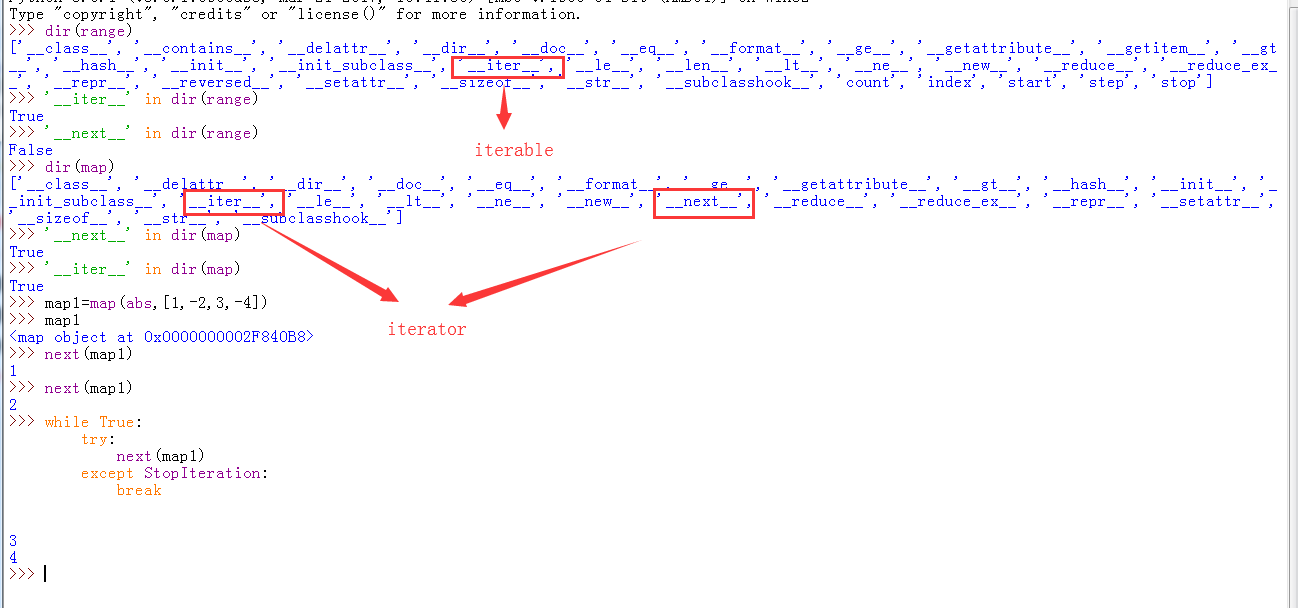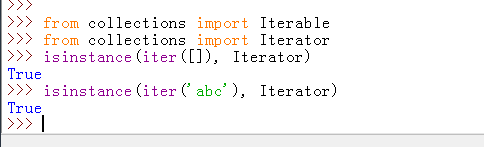• 累计撰写 270 篇文章
• 累计创建 6 个标签
• 累计收到 1 条评论

### 目 录CONTENT# Python的列表推倒式、生成器及迭代器.md2021-08-22 / 0 评论 / 0 点赞 / 4 阅读 / 7,541 字 / 正在检测是否收录...

# Python的列表推倒式、生成器及迭代器

@[toc]

## 一、列表推倒式

1 老母鸡 = ('鸡蛋%s'%i for i in range(10))
2 print(老母鸡)
3 for 蛋 in 老母鸡:
4     print(蛋)
5 g = (i*i for i in range(10))
6 for i in g:
7     print(i)

•  ①把列表解析的[]换成()得到的就是生成器表达式
•  ②列表解析与生成器表达式都是一种便利的编程方式，只不过生成器表达式更加节省内存空间
•  ③Python不但使用迭代器协议，让for循环变得更加通用。大部分内置函数，也是使用迭代器协议访问对象的。

例如sum函数是python的内置函数，该函数使用迭代器协议访问对象，而生成器实现了迭代器协议。

1 sum(i*2 for i in range(4))


### 1.列表推导式

 例一:30以内所有能被3整除的数

1 # 列推导式
2 # [满足条件的元素相关的操作 for 元素 in  可迭代数据类型 if 元素相关的条件]
3 # 30以为所有能被3整除的数字
4 print([i for i in range(30) if i%3 == 0]) # 完整的列表推导式

例二：30以内所有能被3整除的数的平方

1 # 30以为所有能被3整除的平方
2 print([i*i for i in range(30) if i%3 == 0]) # 完整的列表推导式

1 # 找到嵌套列表中名字中含有'e'的所有名字
2 names = [['Tom','Billy','Jefferson','Andrew','Wesley','Steven','Joe'],
3         ['Alice','Jill','Ana','Wendy','Jennifer','Sherry','Eva']]
4 ret = [name for lst in names for name in lst if name.count('e') == 2]
5 print(ret)


### 2.字典推导式

例一:将一个字典的key和value对调

1 # 将一个字典的key和value对调
2 mcase = {'a':10,'b':34}
3 mcase_frequency = {mcase[k]:k for k in mcase}
4 print(mcase_frequency)

1 # 合并大小写对应的value值，将key统一成小写
2 # {'a':10+7,'b':34,'z':3}
3 mcase = {'a':10,'b':34,'A':7,'Z':3}
4 mcase_frequency = {k.lower():mcase.get(k.lower(),0)+mcase.get(k.upper(),0) for k in mcase}


### 3.集合推导式

例子：计算列表中每个值的平方，自带去重复的功能

1 # 集合推导式 自带去重功能
2 squared = {x**2 for x in [1,-1,2]}
3 print(squared)


## 二、生成器

•  通过列表生成式，我们可以直接创建一个列表。但是，受到内存限制，列表容量肯定是有限的。而且，创建一个包含100万个元素的列表，不仅占用很大的存储空间，
•  如果我们仅仅需要访问前面几个元素，那后面绝大多数元素占用的空间都白白浪费了
•  所以，如果列表元素可以按照某种算法推算出来，那我们是否可以在循环的过程中不断推算出后续的元素呢？
•  这样就不必创建完整的list，从而节省大量的空间。在Python中，这种一边循环一边计算的机制，称为生成器：generator。
•  要创建一个generator，有很多种方法。第一种方法很简单，只要把一个列表生成式的[]改成()，就创建了一个generator

### 生成器的创建方式

1. 类似于列表生成式
2. 函数中使用yield关键字

1. 函数调用之后就得到了一个生成器，
2. return 在生成器里，代表生成器的中止，直接报错
3. yield的作用是：返回数据 ，并冻结（暂停）当前的执行过程 ，下一次从暂停处继续执行

### 类似于列表生成式创建

L = [x * x for x in range(10)]
gen_L = (x * x for x in range(10))  # 生成器存放计算公式

print(L)
print(gen_L)
print(next(gen_L))  # 取值
print(next(gen_L))

"""
[0, 1, 4, 9, 16, 25, 36, 49, 64, 81]
<generator object <genexpr> at 0x0000000001DFDF10>  generator 就是生成器的意思
0
1
"""

•  generator保存的是算法，每次调用next(g)就计算出g的下一个元素的值，直到计算到最后一个元素，没有更多的元素时，抛出StopIteration的错误，不调next函数并不会生层元素，就会节省内存，使用for循环可以解决异常
g = (x * x for x in range(10))
for n in g:
print(n)

•  generator非常强大。如果推算的算法比较复杂，用类似列表生成式的for循环无法实现的时候，还可以用函数来实现。
def fib(max):
n, a, b = 0, 0, 1
while n < max:
print('before yield')
yield b  # 把函数的执行过程冻结在这一步，并且把b的值 返回给外面的next()
print(b)
a, b = b, a + b
n = n + 1
return 'done'

f = fib(15)  # turn function into a generator
next(f)  # first time call next()  next 唤醒冻结的函数执行过程，继续执行，直到遇到下一个yield
next(f)  # first time call next()

"""
before yield
1
before yield
"""


a, b = 0, 1
a, b = b, a + b
print(a, b)
a, b = 0, 1
t = a
a = b
b = t + b
print(a, b)

"""
1 1
1 1
"""


### 函数方式创建

def range2(n):

count = 0
while count < n :
print(count)
stop_flag = yield count #中断并返回

return count
if stop_flag == 'stop':
print('stop iteration...')
break
count += 1

b = range2(5) # 得到生成器
next(b)
next(b)
"""
0
Traceback (most recent call last):
File "C:/Users/jingjing/PycharmProjects/py3Project", line 53, in <module>
next(b)
StopIteration: 0
"""


### 生成器常用方法

next()  # 唤醒生成器并继续执行
send("stop")
"""
1. 唤醒并继续执行
2. 发送一个信息到生成器内部

"""


### send方法使用举例

send方法第一次调用必须传递一个None参数，后续才可以传递自定义参数

def range2(n):

count = 0
while count < n:
print('count', count)
count += 1
sign = yield count  # return
if sign == 'stop':
print("---sign", sign)
break
print('sin...', sign)
return 3333

new_range = range2(3)

next(new_range)
new_range.send(None)
new_range.send("stop")
"""
count 0
sin... None
count 1
---sign stop
Traceback (most recent call last):
File "C:/Users/jingjing/PycharmProjects/py3Project.py", line 22, in <module>
new_range.send("stop")
StopIteration: 3333
"""


### 生产者与消费者问题

import time

def consume(name):
print("%s 准备吃包子啦!" % name)
while True:
y = yield
print("包子[%s]来了,被[%s]吃了!" % (y, name))

def producer(name):
c = consume(name)
next(c)  # 函数执行到yield
for i in range(1, 3):
time.sleep(1)
print("做了{}个包子!".format(i))
c.send(i)  # 把i传到yield

producer('qian')

"""
qian 准备吃包子啦!

"""


### 日志记录

def logger(filename):
"""
日志方法
:param filename: log filename
:param channel:  输出的目的地，屏幕(terminal)，文件(file)，屏幕+文件(both)
:return:
"""
print('start logger')
while True:
msg = yield
print("msg", msg)

l = logger('USER.TXT')
l.__next__()

l.send('hi')
l.send('hi,file')


### 计算移动平均值

# 必须先用next再用send
def average():
total=0 #总数
day=0 #天数
average=0 #平均数
while True:
day_num = yield average   #average=0
print('average', average)
total += day_num
day += 1
average = total/day
avg=average() #直接返回生成器
next(avg)#激活生成器，avg.send(None),什么都不传的时候send和next的效果一样
print(avg.send(10))
print(avg.send(20))#send   1.传值 2.next
print(avg.send(30))
"""
average 0
10.0
average 10.0
15.0
average 15.0
20.0
"""


### 带装饰器的计算移动平均值

# 让装饰器去激活
def wrapper(func):
def inner(*args, **kwargs):
print('execute wrapper')
a = func(*args, **kwargs)
next(a)
return a
return inner

@wrapper
def average():
total=0 #总数
day=0 #天数
average=0 #平均数
while True:
day_num = yield average   #average=0
print('average', average)
total += day_num
day += 1
average = total/day

avg = average()
print(avg.send(10))
print(avg.send(20))#send   1.传值 2.next
print(avg.send(30))
"""
execute wrapper
average 0
10.0
average 10.0
15.0
average 15.0
20.0
"""


### yield from

def gen1():
for c in 'AB':
yield c
for i in range(3):
yield i
print(gen1())
print(list(gen1()))

def gen2():
yield from 'AB'  # 相当于  for c in 'AB': yield c
yield from range(3)
print(gen2())
print(list(gen2()))


### 处理异常

def fib(max):
n, a, b = 0, 0, 1
while n < max:
print('before yield')
yield b  # 把函数的执行过程冻结在这一步，并且把b的值 返回给外面的next()
a, b = b, a + b
n = n + 1
return 'done'

g = fib(6)
while True:
try:
x = next(g)
print('g:', x)
except StopIteration as e:
print('Generator return value:', e.value)
break


## 三、迭代器

### 可迭代对象与迭代器

1. 迭代：可以将某个数据集内的数据“一个挨着一个的取出来”，就叫做迭代。就像for循环一样取值。

2. 可迭代协议：可以被迭代要满足要求的就叫做可迭代协议。内部实现了__iter__方法

3. iterable：可迭代的------对应的标志

4. 字符串、列表、元组、字典、集合都可以被for循环，说明他们都是可迭代的

5. 可以使用isinstance()判断一个对象是否是Iterable对象

6. 可以被next函数调用并且返回下一个值的对象叫迭代器

from collections import Iterable

print(isinstance([], Iterable))
print(isinstance(123, Iterable))
print(isinstance('345', Iterable))
# """
# True
# False
# True
# """


### 可迭代和迭代器区别

#### 1. 区别：

•  可迭代的结构并不代表一定是一个迭代器，如：列表是可迭代的但不是迭代器；生层式是可迭代的也是迭代器
•  可以通过系统函数iter（）将一个可迭代的结构变成一个迭代器

#### 2.迭代器：

•  迭代器协议：内部实现了__iter__，__next__方法。

#### 4.相同点与不同点：

•  可迭代和迭代器的相同点：都可以用for循环
•  可迭代和迭代器的不同点：就是迭代器内部多实现了一个__next__方法

#### 5.判断迭代器和可迭代的方法

•  第一种：判断内部是不是实现了__next__方法
•  print('next' in dir(range(12))) #查看'next'是不是在range()方法执行之后内部是否有__next__
•  print('iter' in dir(range(12))) #查看'next'是不是在range()方法执行之后内部是否有__next__
•  第二种：
•  Iterable 判断是不是可迭代对象
•  Iterator 判断是不是迭代器
from collections import Iterable
from collections import Iterator

#比如给一个字符串
s='abc'
print(isinstance(s,Iterable))#isinstance判断类型的
print(isinstance(s,Iterator))


### 判断range函数和map函数### 深入了解Iterator对象•  这是因为Python的Iterator对象表示的是一个数据流，Iterator对象可以被next()函数调用并不断返回下一个数据，
•  直到没有数据时抛出StopIteration错误。可以把这个数据流看做是一个有序序列，但我们却不能提前知道序列的长度，
•  只能不断通过next()函数实现按需计算下一个数据，所以Iterator的计算是惰性的，只有在需要返回下一个数据时它才会计算。
•  Iterator甚至可以表示一个无限大的数据流，例如全体自然数。而使用list是永远不可能存储全体自然数的。

### 迭代器小结

•  凡是可作用于for循环的对象都是Iterable类型；
•  凡是可作用于next()函数的对象都是Iterator类型，它们表示一个惰性计算的序列；
•  集合数据类型如list、dict、str等是Iterable但不是Iterator，不过可以通过iter()函数获得一个Iterator对象。
•  Python3的for循环本质上就是通过不断调用next()函数实现的，例如：
for x in [1, 2, 3, 4, 5]:
pass

# 首先获得Iterator对象:
iter_object  = iter([1, 2, 3, 4, 5])
# 循环:
while True:
try:
# 获得下一个值:
x = next(iter_object)
except StopIteration:
# 遇到StopIteration就退出循环
break


0